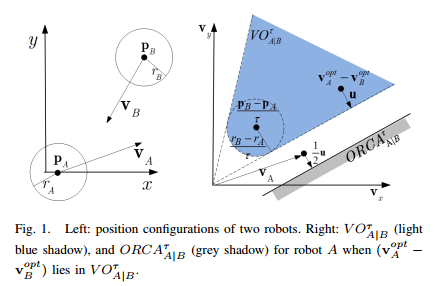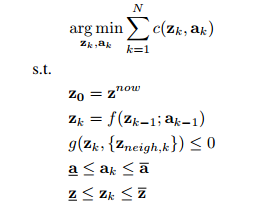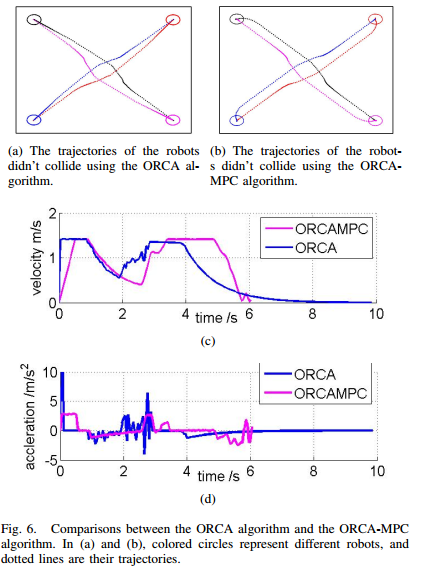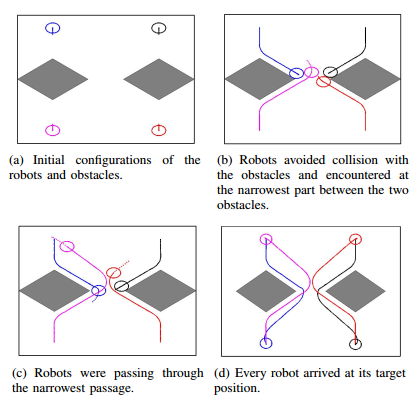IROS 2017
Decentralized Navigation of Multiple Robots Based on ORCA and Model Predictive Control
Hui Cheng, Qiyuan Zhu, Zhongchang Liu, Zeyu Jiang, and Liang Lin
IROS 2017

Abstract

This paper presents a decentralized strategy for reciprocal collision-free navigation of multiple robots. This strategy combines the Optimal Reciprocal Collision Avoidance (ORCA) algorithm and Model Predictive Control (MPC). Each robot applies the decentralized ORCA algorithm to compute the collision avoidance velocities with respect to its neighbors. The derived velocities serve as constraints of a MPC problem whose solution provides the optimal control input that can ensure optimal motion of the robot. The states predicted from the robots’ dynamic models are used in the ORCA algorithm to compute the ORCA velocity regions in future steps. This ORCA MPC combined approach doesn’t need apriori the preferred velocity of each robot in comparison to the traditional ORCA algorithm and its existing variants. Moreover, the convexity of the ORCA velocity region is retained even after incorporating the dynamic models of the robots. Simulation results illustrate the effectiveness of the proposed method, and show that this new algorithm can reduce velocity vibrations compared to the traditional ORCA algorithm.

Introduce

The model predictive control (MPC) method can predict future trajectories of a system subject to the system dynamics and various constraints. So, it is an attractive approach to controlling robot motions as well as avoiding obstacles, and has been used recently in the navigation problems of a single robot . Motivated by these results, an ORCA-MPC combined approach is proposed for multi-robot navigation. This approach uses the ORCA algorithm to generate the set of permitted velocities, constrained by which a MPC problem is solved to generate the optimal control input by minimizing a cost function that penalizes the control cost and the distance to the target position. Since the cost function is irrelevant to the preferred velocity, the ORCA-MPC algorithm doesn’t need an additional procedure to compute the preferred velocity. Moreover, each robot’s trajectory and velocity are optimized and smooth. Another advantage of the ORCAMPC combined approach is that the permitted velocity set generated by the ORCA algorithm is still convex although the dynamic models of the robots are introduced.

The MPC problem takes the following form :ExperimentSimulation results in Fig. 6(b) and 6(a) show that both the ORCA algorithm and the ORCA-MPC algorithm can navigate the robots to their target positions without causing collisions. Using these two algorithms, Fig. 6(c) shows the corresponding two velocity trajectories for one of the four robots, in which the speed fluctuations around time 1s to 3s indicates that the robot are changing its speed to avoid collisions with other robots. In this period, the velocity curve using the ORCA-MPC algorithm is much smoother than that using the ORCA algorithm. We can draw the same conclusion for the acceleration curves in Fig. 6(d). We can also see from this figure that the accelerations (which server as control inputs) using the ORCA algorithm may exceed the upper bound, and they vibrated so heavily and frequently that severe wear and tear can be caused on the control devices.Navigation of four robots using the ORCA-MPC algorithm in workspace that contains static obstacles .

References

J. Van Den Berg, J. Snape, S. J. Guy, and D. Manocha, “Reciprocal collision avoidance with acceleration-velocity obstacles,” in IEEE International Conference on Robotics and Automation, 2011, pp. 3475–3482 .

A. Domahidi, A. U. Zgraggen, M. N. Zeilinger, M. Morari, and C. N. Jones, “Efficient interior point methods for multistage problems arising in receding horizon control,” in IEEE Conference on Decision and Control, 2012, pp. 6 –674.